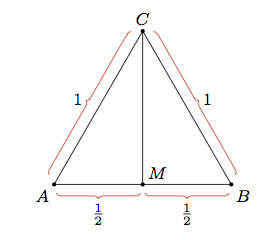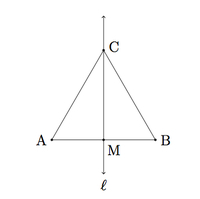# Finding the Area of an Equilateral Triangle

Alignments to Content Standards: G-CO.C.10 G-SRT.C

Below is an equilateral triangle whose side lengths are each $1$ unit:Find the area of $\triangle ABC$.

## IM Commentary

This tasks examines how to calculate the area of an equilateral triangle using high school geometry, further developing ''8.G Areas of geometric shapes with the same perimeter'' which performs the same calculation at a level appropriate for middle school. In order to use the formula for the area of a triangle $$\text{Area} = \frac{1}{2} \text{base} \times \text{height}$$ we can use any side of the triangle as a base and the key will be finding the height of the triangle with that base. In practice this means finding the line passing through the third vertex meeting the base in a right angle.

Two different solutions are presented. The first finds the appropriate altitude using triangle congruence. The second uses trigonometry. It is important for students to understand both solutions and the teacher should emphasize that the first approach focuses on side lengths in order to construct an altitude for the triangle while the second approach focuses on angles.

The task is mainly intended for instructional purposes as both solutions are important. It would also be valuable to compare the arguments here to the more intuitive ones presented in the eighth grade problem indicated above.

## Solutions

Solution: 1 Congruent Triangles

Labelling the vertices of the triangle $A$, $B$, and $C$, let $M$ be the midpoint of side $\overline{AB}$ as pictured below:Triangles $AMC$ and $BMC$ are congruent as we can show with $SSS$:

• $\overline{AM}$ is congruent to $\overline{BM}$ since $M$ is the midpoint of $\overline{AB}$,
• $\overline{AC}$ is congruent to $\overline{BC}$ because triangle $ABC$ is equilaterial,
• $\overline{MC}$ is congruent to $\overline{MC}$.

Angles $CMB$ and $CMA$ are corresponding angles of the congruent triangles $AMC$ and $BMC$ and so are congruent. Since these two angles make a line and are congruent they must each be right angles. We can therefore apply the Pythagorean theorem to $\triangle AMC$ to find $|MC|$: $$|MC|^2 + |AM|^2 = |AC|^2.$$ We know that $|AM| = \frac{1}{2}$ since $|AB| = 1$ and $M$ is the midpoint of $|AB|$. We were also given that $|AC| = 1$. Plugging this information into formula above we get $$|MC|^2 + \frac{1}{4} = 1.$$ So $|MC|^2 = \frac{3}{4}$ and $$|MC| = \frac{\sqrt{3}}{2}.$$ Now we have found the height of triangle $ABC$, using $\overline {AB}$ as a base, and so the area of triangle $ABC$ is $$\frac{1}{2}\times 1 \times \frac{\sqrt{3}}{2} = \frac{\sqrt{3}}{4}.$$

Solution: 2 Trigonometric ratios

We label the triangle vertices $A,B,C$ as in the previous solution. Suppose line $\ell$ bisects angle $A$, as in the picture below, and let $M$ be the point of intersection of $\ell$ with $\overline{AB}$:Since $\triangle ABC$ is equilateral, we know that $m(\angle A) = m(\angle B) = m(\angle C) = 60$. We have $m(\angle ACM) = m(\angle BCM) = 30$ since $\overrightarrow{CM}$ bisects angle $C$. The sum of the three angles in $\triangle ACM$ is $180^\circ$. Since $m(\angle CAM) = 60$ and $m(\angle ACM) = 30$ this means that $m(\angle CMA) = 90$. So $\overline{MC}$ is an altitude for triangle $ABC$, using $\overline{AB}$ as a base. To find the height of the altitude we can use trigonometry: $$\sin{\angle A} = \frac{|MC|}{|AC|}.$$ Since $\sin{60^\circ} = \frac{\sqrt{3}}{2}$ and $|AC| = 1$ we have $$|MC| = \frac{\sqrt{3}}{2}.$$ Since $|AB| = 1$ we find that the area of $\triangle ABC$ is $$\frac{1}{2} \times 1 \times \frac{\sqrt{3}}{2} = \frac{\sqrt{3}}{4}.$$